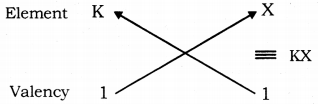# From the following elements: 4Be , 9F , igK , 20Ca

From the following elements:
4Be, 9F, 19K, 20Ca
(i) Select the element having one electron in the outermost shell.
(ii) Two elements of the same group.

Write the formula and mention the nature of the compound formed by the union of 19K and element X (2, 8, 7).

(i) Among the given elements, 19K has one electron in the outermost shell. Its electronic configuration is 2, 8, 8, 1.

(ii) The elements 4Be and 20Ca belong to the same group, that is, Group 2. They both have two electrons each in their outermost shells.
Electronic configuration of 4Be is 2, 2
Electronic configuration of 20Ca is 2, 8, 8, 2.
Atomic number of element X = 17
Electronic configuration of element X is 2, 8, 7.
Number of valence electrons in X = 7
Valency of X = 8 - 7 = 1
Atomic number of K = 19
Electronic configuration of K is 2, 8, 8, 1.
Number of valence electrons in K = 1
Valency of K = 1Therefore, the formula of the compound formed when K and element X combine is KX is of ionic nature.